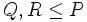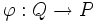# Saturated fusion system

This is a variation of group|Find other variations of group | Read a survey article on varying group

## Definition

Let$P$ be a group of prime power order, say a finite$p$-group, for a prime$p$. A saturated fusion system$\mathcal{F}$ on$P$ is a category on$P$ (in the sense of a category on a finite p-group) with the following properties:

Condition name Qualitative description of condition Condition details
contains inner fusion system This condition makes sure that the obvious homomorphisms are included For any subgroups$Q, R \le P$, all injective homomorphisms from$Q$ to$R$ that arise as restrictions of inner automorphisms of$P$, are present in$\mathcal{F}$. In other words, the inner fusion system on$P$ is a subcategory of$\mathcal{F}$.
Sylow axiom This condition is a purely global condition, i.e., it gives information only about automorphisms at the global level The inner automorphisms of$P$ form a Sylow subgroup of the group of automorphisms of$P$ in$\mathcal{F}$.
Extension axiom This condition links up the local and the global Call a subgroup$R$ of$P$ fully normalized by$\mathcal{F}$ if$|N_P(Q)| \le |N_P(R)|$ for any$Q \cong R$ where$\cong$ is isomorphism in the category$\mathcal{F}$.
Also define, for any morphism$\varphi:Q \to P$ in$\mathcal{F}$:$N_\varphi = \{ y \in N_P(Q) \mid \exists z \in N_P(\varphi(Q)), \varphi(yuy^{-1}) = z\varphi(u)z^{-1} \ \forall u \in Q \}$
Then the statement of the extension axiom is:
Every morphism$\varphi:Q \to P$ such that$\varphi(Q)$ is fully$\mathcal{F}$-normalized, extends to a morphism$\psi:N_\varphi \to P$.

## Terminological note

Some people use the term fusion system for what is defined here as a saturated fusion system. Others use fusion system to mean a category on a finite p-group that contains the inner fusion system. People in both terminological camps are usually interested only in saturated fusion systems when they talk of fusion systems.

There are multiple conventions on whether the term fusion system should refer to saturated fusion system or to a weaker notion. For our purposes, we will mostly be interested in saturated fusion systems, because these mimic/generalize situations where the$p$-group is a$p$-Sylow subgroup of some finite group. Unless otherwise specified, we are referring to saturated fusion systems when we talk about fusion systems.